• MATLAB 多元线性回归 含例题数据，和可运行的MATLAB代码。MATLAB 多元线性回归 含例题数据，和可运行的MATLAB代码。
• 主要用于数学建模（Matlab）的学习，下载下来换成你的数据就可以用了。
• 多元线性回归:在回归分析中，如果有两个或两个以上的自变量，就称为多元回归。事实上，一种现象常常是与多个因素相联系的，由多个自变量的最优组合共同来预测或估计因变量，比只用一个自变量进行预测或估计更有效，...测绘工程
• MATLAB 多元线性回归（regress） 语法 b = regress(y,X) [b,bint] = regress(y,X) [b,bint,r] = regress(y,X) [b,bint,r,rint] = regress(y,X) [b,bint,r,rint,stats] = regress(y,X) [___] = regress(y,X,alpha) ...
MATLAB 多元线性回归（regress）
语法
b = regress(y,X) [b,bint] = regress(y,X) [b,bint,r] = regress(y,X) [b,bint,r,rint] = regress(y,X) [b,bint,r,rint,stats] = regress(y,X) [___] = regress(y,X,alpha)
说明
b = regress(y,X) returns a vector b of coefficient estimates for a multiple linear regression of the responses in vector y on the predictors in matrix X. To compute coefficient estimates for a model with a constant term (intercept), include a column of ones in the matrix X. b=回归（y，X）返回一个系数估计向量b，用于矩阵X中预测器上向量y中的响应的多元线性回归。若要计算具有常数项（截距）的模型的系数估计，请在矩阵X中包含一列。
[b,bint] = regress(y,X) also returns a matrix bint of 95% confidence intervals for the coefficient estimates. [b，bint]=回归（y，X）也返回系数估计的95%置信区间的矩阵bint。
[b,bint,r] = regress(y,X) also returns an additional vector r of residuals. [b，bint，r]=回归（y，X）还返回一个残差向量r。
[b,bint,r,rint] = regress(y,X) also returns a matrix rint of intervals that can be used to diagnose outliers. [b，bint，r，rint]=回归（y，X）还返回一个可用于诊断异常值的间隔矩阵。
[b,bint,r,rint,stats] = regress(y,X) also returns a vector stats that contains the R2 statistic, the F-statistic and its p-value, and an estimate of the error variance. The matrix X must include a column of ones for the software to compute the model statistics correctly. [b，bint，r，rint，stats]=回归（y，X）还返回一个向量统计，其中包含R2统计量、F-统计量及其p值以及误差方差的估计。矩阵X必须包含一列，以便软件正确计算模型统计数据。
[___] = regress(y,X,alpha) uses a 100*(1-alpha)% confidence level to compute bint and rint. Specify any of the output argument combinations in the previous syntaxes. [___]=回归（y，x，alpha）使用100*（1-alpha）%置信度来计算bint和rint。在前面的语法中指定任何输出参数组合。
展开全文• 基于matlab的各种多元统计分析模型源代码-多元线性回归MultiLineReg.rar 本人为了获得更多资源共享的权限，只好吐血奉献自己一年来收集和改写的matlab源程序，部分为原创；里面包含有主成分分析、岭回归分析、因子...
• 1.线性回归: regress 顾名思义，就是一元多元方程的的拟合，y=c1*x1+c2*x2....或者y=c1*x1^2+c2*x2^2+c3*x1*x2....等等形式 [b,BINT] = regress(Y,X) [b,BINT,R] = regress(Y,X) [b,BINT,R,RINT] = regress(Y,X) ...
1.线性回归:
regress 顾名思义，就是一元多元方程的的拟合，y=c1*x1+c2*x2....或者y=c1*x1^2+c2*x2^2+c3*x1*x2....等等形式
[b,BINT] = regress(Y,X)
[b,BINT,R] = regress(Y,X)
[b,BINT,R,RINT] = regress(Y,X)
[b,BINT,R,RINT,STATS] = regress(Y,X)
[b,BINT,R,RINT,STATS] = regress(Y,X,ALPHA)

b [c1 c2 c3.....]
BINT 回归系数的估计区间 B的95%的置信区间矩阵，Bint 置信区间不大，说明有效性较好；若含零点，说明结果无效。
R 残差（因变量的真实值减去估计值）
RINT 置信区间

STATS：向量，STATS中的4个值分别为：R2（判定系数），F（总模型的F测验值），P（总模型F的概率值P(F>Fz)），MSq（离回归方差或误差方差的估计值）

判定系数(the Coefficient of the Determination)R2：是判断回归模型拟合程度的一个指标，其取值范围为[0, 1]；判定系数越大说明回归模型的拟合程度越高，回归方程越显著。

F>F(1-α)(k, n-k-1)时拒绝H0，F越大，说明回归方程越显著。
与F对应的概率P<α时拒绝H0，回归模型成立。

MSq：由于最小二乘法中不求误差方差σ2，其误差平方和Msq定义为SSR/自由度
funcPara=[ones(size(y,2))' x1' x2']; [b,bint,r,rint,stats] =regress(y‘,funcPara); %注意： 函数内部使用的向量都要为列向量
2.拟合ployfit
polyfit函数基于最小二乘法，使用的基本格式为：
p = polyfit(x,y,n)
[p,S] = polyfit(x,y,n)
[p,S,mu] = polyfit(x,y,n)

其中每个命令中的n为多项式拟合的次数，当n为1时，即为一次拟合（很多情况下等价于一元线性回归）。p是n+1维参数向量p(1)，p(2)….那么拟合后对应的多项式即为p(1)*x^n + p(2)*x^(n-1) +…+ p(n)*x + p(n+1)。S是规模为1×1的结构数组，包括R（系数矩阵的QR分解的上三角阵），df（自由度），normr（拟合误差平方和的算术平方根）。
例子1：拟合Sellmeier
% -----------------Sellmeier
wavelength=[0.21000 0.37830 1.0330 2.2120 3.4700 4.1260 4.4220 4.7390 5.4430 6.0390 6.4720 6.7000];%波长
n=[1.5383576204905 1.4727046797948 1.4500069615101 1.4348196176837 1.4067782146466 1.3841208059058 1.3713701305288 1.3555262189157 1.3096384003386 1.2537289561387 1.19732567716307 1.1596494139777];%折射率
f=@(P,w)(sqrt(1+P(1)*w.^2./(w.^2-P(2))+P(3)*w.^2./(w.^2-P(4))+P(5)*w.^2./(w.^2-P(6))));%设置函数样式
P=[1;0;1;0;1;100]; %预估参数值
P=nlinfit(wavelength,n,f,P);%拟合参数值
wave=0.21:0.001:6.7;%横轴扩展
n1=f(P,wave);%纵轴计算
figure(1);
plot(wave,n1,wavelength,n,'o');
xlabel('Wavelength');
ylabel('n');
例子2：拟合正弦函数，详细的可以参见：https://ww2.mathworks.cn/help/matlab/ref/polyfit.html
x = linspace(0,4*pi,10);
y = sin(x);
p = polyfit(x,y,7);
x1 = linspace(0,4*pi);
y1 = polyval(p,x1);
figure
plot(x,y,'o')
hold on
plot(x1,y1)
hold off
结论：都可以进行线性回归拟合，但是第一种更直观，且能得到更多的信息，第二种的话使用起来比较简单。
展开全文• 基于MATLAB的多元非线性回归模型,matlab多元线性回归模型实例,matlab源码
• 关于如何使用Matlab构建多元线性回归模型在数学建模中有许多情况需要用到多远非线性回归数学建模
• 多元线性回归及显著性检验Matlab程序完美版 一说明 1本程序是硕士教材数理统计杨虎刘琼钟波 编著 例4.4.1P133Matlab编程解答程序教材上例题只做了回归方程显著性分析和一次回归系数显著性分析剔除x1后没有再检验x2和...
• matlab偏最小二乘法代码，整理各种线性和非线性回归，参考网上资料
• %%1、bint表示回归系数区间估计 %2、r表示残差 %3、rint代表置信区间 %4、stas表示用于检验回归模型的统计量，有三个数值 r^2 F 与F对应的概率P 例如p<0.05 残差95% % r^2越接近于1，回归方程越显著 %alpha表示...
1、案例
%%1、bint表示回归系数区间估计可参考http://www.360doc.com/content/11/0801/20/2537127_137246007.shtml
%2、r表示残差
%3、rint代表置信区间
%4、stas表示用于检验回归模型的统计量，有三个数值 r^2 F 与F对应的概率P 例如p<0.05 残差95%
%   r^2越接近于1，回归方程越显著
%alpha表示显著水平

%%
x=[143 144 145 147 148 150 153 154 155 156 157 158 159 160 161 162]';
X=[ones(16,1),x];
Y=[87 85 88 91 92 90 93 95 98 98 97 95 97 99 100 102]';
[b,bint,r,rint,stats]=regress(Y,X)
t=1:16;
%%
figure(1);
y_fitting=X(t,:)*b;
plot(t,y_fitting,'r-',  t,Y(t,:),'b-', t,abs(y_fitting-Y(t,:)),'k-');
legend('红--拟合值','蓝--实际值','黑--误差值');
text(3,50,strcat('相关系数R=',num2str(stats(1,1 ))));
text(7,50,strcat('F=',num2str(stats(1,2))));
text(9,50,strcat('P=',num2str(stats(1,3 ))));
nhfcs1=strcat('拟合方程式',num2str(b(1,1)),'+',num2str(b(2,1)),'*X1');
text(11,50,nhfcs1);
%
%功能 在当前轴中创建text对象。函数text是创建text图形句柄的低级函数。可用该函数在图形中指定的位置上显示字符串。

%用法 text(x,y,'string')在图形中指定的位置(x,y)上显示字符串string

%text(x,y,z,'string') 在三维图形空间中的指定位置(x,y,z)上显示字符串string
%
title('线性回归曲线拟合结果');
xlabel('样本点');
ylabel('分数');

%%
figure(2);
ul=rint(:,1);
I1=rint(:,2);
plot(t,I1,'b-', t,r,'R*',  t, ul,'g-');
legend('蓝色--残差95%置信区间上限','红--残差值','绿--残差95%置信区间下限');
xlabel('样本值');
ylabel('残差值');
figure(3)
rcoplot(r,rint);   %残差分析，作残差图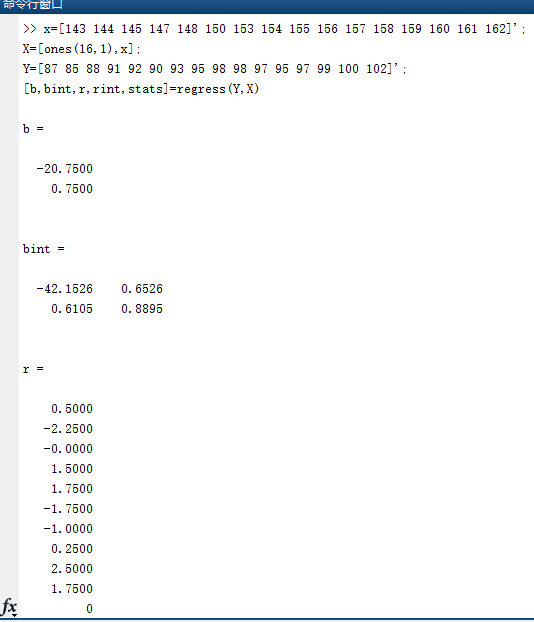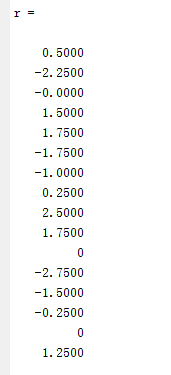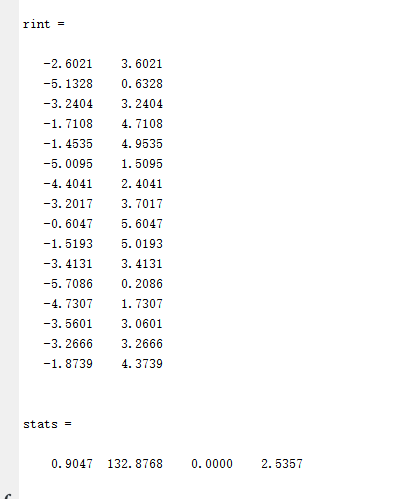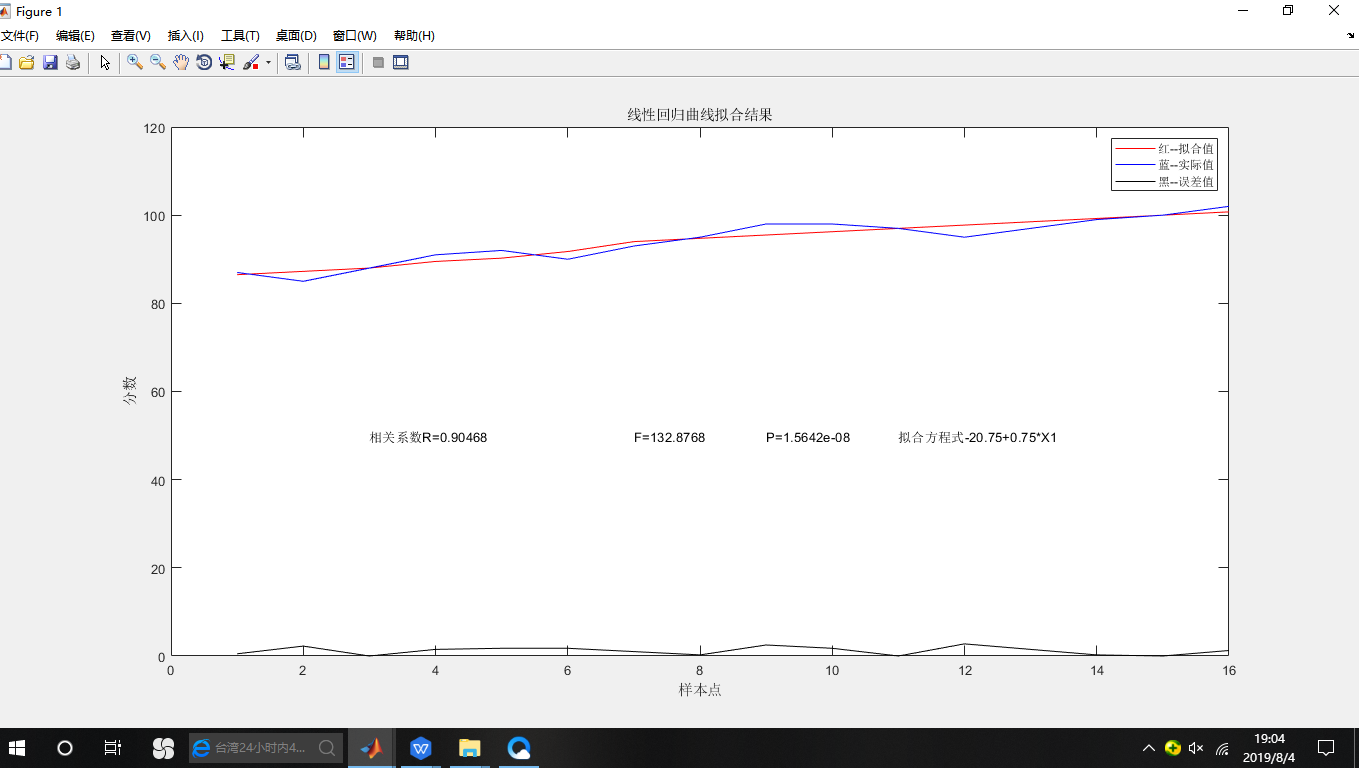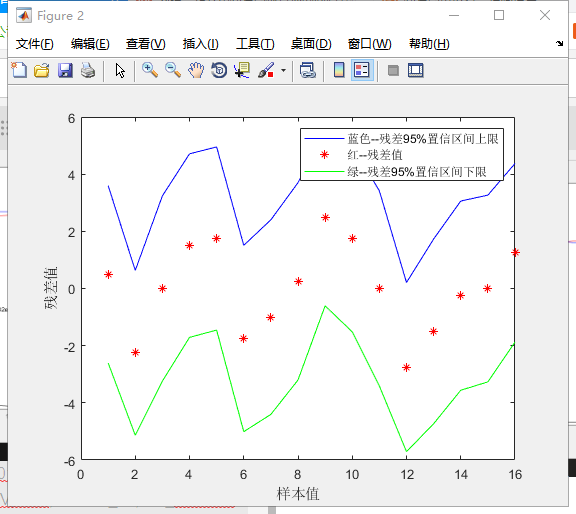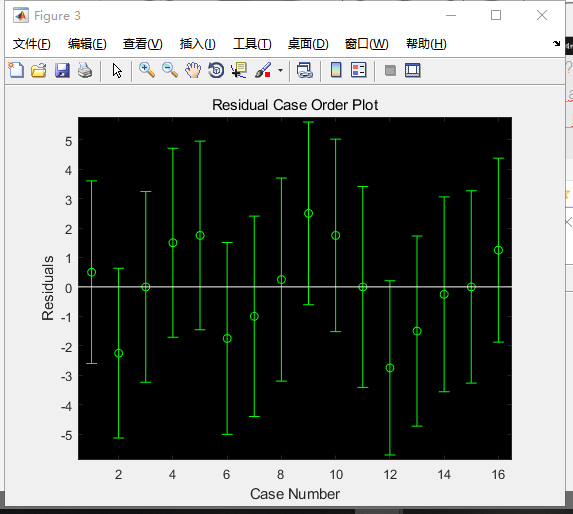2、残差图(Residual Plots)
我们可以用残差图来估计观察或预测到的误差error(残差residuals)与随机误差(stochastic error)是否一致。用一个丢骰子的例子最好理解了。当你丢出去一个六面的骰子时，你不应该能够预测得到哪面点数向上。然而，你却可以评估在一系列投掷后，正面向上的数字是否遵循一个随机模式，你自己心中就会想象出一个随机散布的残差图。如果，有人背着你对骰子做了点手脚，让六点更频繁的出现向上，这时你心中的残差图看上去就似乎有规律可循，从而不得不修改心中的模型，让你狐疑骰子一定有问题。 相同的原则也适用于回归模型。你不应该能够预测任何给定的观察或预测结果的错误(或者说差别)。你需要确定残差是否与随机误差相互呈现一致性，就像丢骰子一样，残差若整体呈现“很古怪”的模式，你就需要回头修改你的回归模型了。上面“古怪”究竟怎么看呢？看下文。

%clc
%clear
%%
%目标函数：y=Ax1^2+Bx2^2+Cx1+Dx2+Ex1*x2+F  （这是一个二次函数，两个变量，大写的字母是常数）
%导入数据
y=[7613.51  7850.91  8381.86  9142.81 10813.6 8631.43 8124.94 9429.79 10230.81 10163.61 9737.56 8561.06 7781.82 7110.97]';
x1=[7666 7704 8148 8571 8679 7704 6471 5870 5289 3815 3335 2927 2758 2591]';
x2=[16.22 16.85 17.93 17.28 17.23 17 19 18.22 16.3 13.37 11.62 10.36 9.83 9.25]';
X=[ones(size(y)) x1.^2 x2.^2 x1 x2 x1.*x2];
%开始分析
[b,bint,r,rint,stats] = regress(y,X)
scatter3(x1,x2,y,'filled') %scatter可用于画散点图a
hold on
%%
%拟合，三维视图显示
hold on  %不要清除计算数据，在刚刚那副散点图上接着画
x1fit = min(x1):100:max(x1);   %设置x1的数据间隔
x2fit = min(x2):1:max(x2);     %设置x2的数据间隔
[X1FIT,X2FIT] = meshgrid(x1fit,x2fit);  %生成一个二维网格平面，也可以说生成X1FIT,X2FIT的坐标
YFIT=b(1)+b(2)*X1FIT.^2+b(3)*X2FIT.^2+b(4)*X1FIT+b(5)*X2FIT+b(6)*X1FIT.*X2FIT;    %代入已经求得的参数，拟合函数式
mesh(X1FIT,X2FIT,YFIT)    %X1FIT，X2FIT是网格坐标矩阵，YFIT是网格点上的高度矩阵
view(10,10)  %改变角度观看已存在的三维图，第一个10表示方位角，第二个表示俯视角。
%方位角相当于球坐标中的经度，俯视角相当于球坐标中的纬度
xlabel('x1') %设置X轴的名称
ylabel('x2') %设置y轴的名称
zlabel('y')  %设置z轴的名称
hold on

%%
figure(2)
rcoplot(r,rint);   %残差分析，作残差图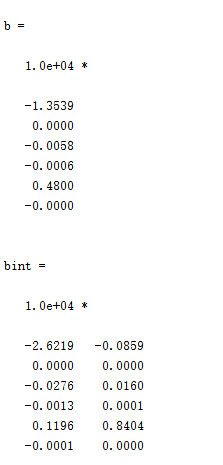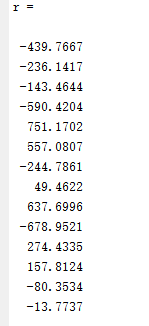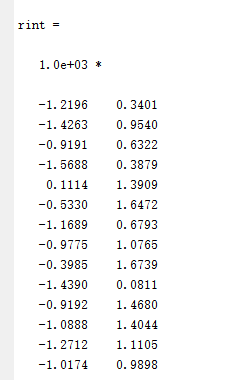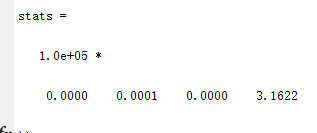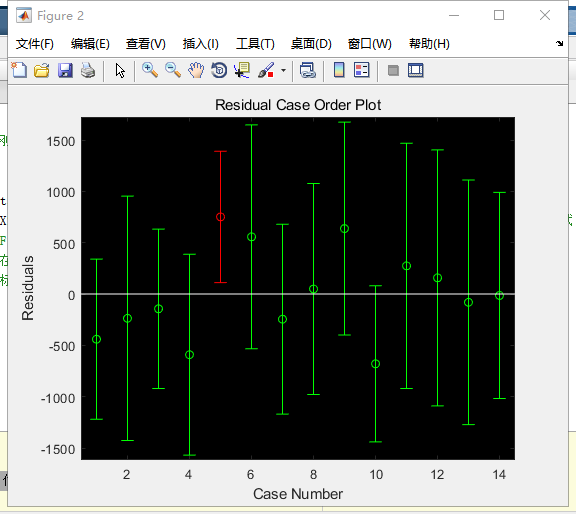https://zhuanlan.zhihu.com/p/20700731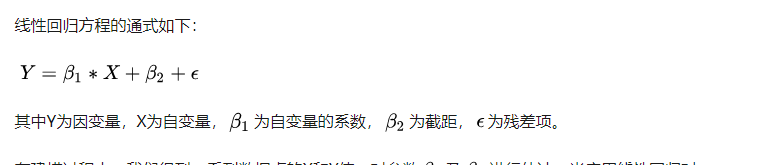3、回归值与残差的残差图编辑
为检验建立的多元线性回归模型是否合适，可以通过回归值与残差的散点图来检验。其方法是画出回归值与普通残差的散点图，或者画出回归值与标准残差的散点图，其图形可能会出现下面三种情况(如图1所示)：
图1（a）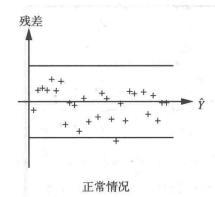图1（b）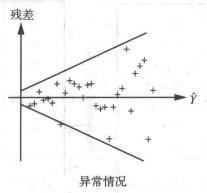对于图1(a)，如果大部分点都落在中间(b)部分，而只有少数几个点落在外边，则这些点对应的样本，可能有异常值存在。
图1（c）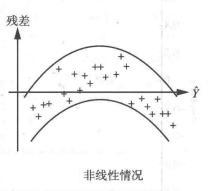展开全文• Matlab多元线性回归分析企业商品价格指数.pdf
•软件开发
• ## matlab多元线性回归

千次阅读 多人点赞 2017-09-10 09:06:11
一、多元线性回归 数学表达式为y=b0+b1*x1+b2*x2+...+bn*xn+E;表达成矩阵的关系为y=X*B+E; matlab 命令为[b,bint,r,rint,stats]=regress(Y,X,alpha); 残差和置信区间可以用rcoplot(r,rint)来画残差图 b=[ ...
一、多元线性回归

数学表达式为y=b0+b1*x1+b2*x2+...+bn*xn+E;
表达成矩阵的关系为y=X*B+E;

matlab 命令为[b,bint,r,rint,stats]=regress(Y,X,alpha);
残差和置信区间可以用rcoplot(r,rint)来画残差图
b=[ b0 b1 b2 ... bn]'; 表示各项的系数
bint：表示各个系数对应的置信空间
r：残差向量
rint：残差向量的置信空间
stats：表示用于检验回归模型的统计量，有四个统计值；可决系数R^2，F值，与F对应的显著性概念p，残差方差S^2
可决系数R^2越接近1，拟合优度越好；
F越大，回归方程越显著 ,S^2越小越好

身高
143
145
146
147
149
150
153
154

腿长
88
85
88
91
92
93
93
95

身高
155
156
157
158
158
160
162
164

腿长<


展开全文• 2 2 Matlab 实现多元回归实例 一一般多元回归 一般在生产实践和科学研究中人们得到了参数 x ?x1,xn ? 和因变量 y 的数据需要求出关系式 y ?...1 时称为一元线性回归当自变量有多个时即 x ? ? x1 , ,xn ? 中 n ?2
• matlab多元参数非线性回归模型代码阅读KDNuggets的注意事项 在从[ KDNuggets ]（）阅读Blog时写了本笔记。 在本简要说明中，它仅包含我感兴趣但并不足够熟悉的术语和主题。 有关完整内容，请参阅原件。 聚类：旨在......

# matlab多元线性回归matlab 订阅